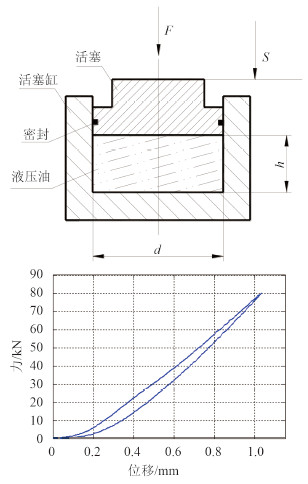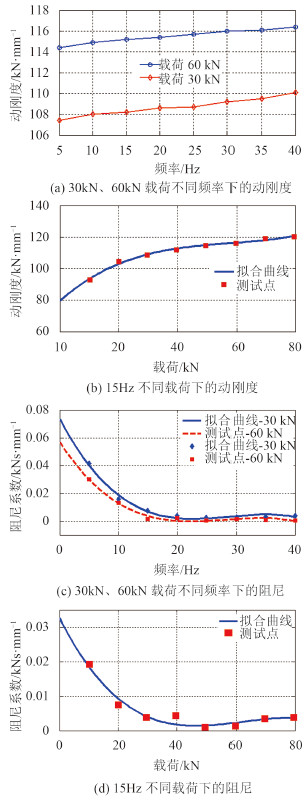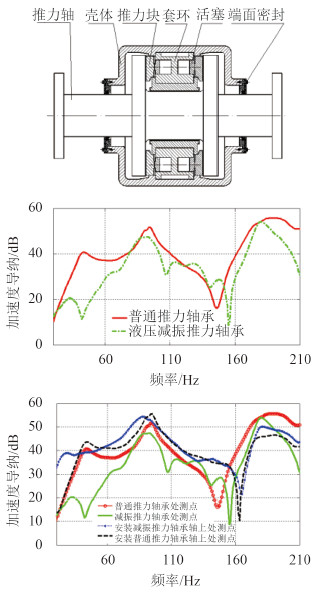﻿ 船舶推力轴承纵向液压减振技术研究
 舰船科学技术2016, Vol. 38Issue (3): 59-62PDF

Research of reducing axial vibration with hydraulic shock absorber in ship's thrust bearing
LIU Wei, WANG Lei, YU Qiang, LIU Yi-jun
China Ship Development and Design Center, Wuhan 430064, China
Abstract: To reducing axial vibration induced by the pulse thrust excitation from propellera hydraulic shock absorber embedded in ship's thrust bearing was introduced. Firstly, the model of propeller-shaft isolation system was set up, and the characteristic of axial vibration was analyzed. Secondly, the dynamic model of hydraulic shock absorber was designed and the computing method of stiffness was proposed. Finally, experiment analysis of thrust bearing with hydraulic shock absorber was developed, the result demonstrated that the designed absorber can reduce the axial vibration effectively, providing a method for the acoustic design of ship's shaft.
Key words: propeller rubber bearing    pressure distribution    structure optimization    finite element analysis
0 引言

Goodwin提出基于液力吸振原理的推进轴系的纵向振动控制技术研究，Dylejko与Merz等在此基础上进行深化，建立声辐射计算模型并提出半主动控制等优化方法。刘义军等开展了碟簧式推力轴承理论与试验研究，取得了较好的减振效果。沈建平等研究了采用舱壁式推力轴承减小轴系纵向振动。张金国等提出应用法兰盘式推力轴承降低纵向激励向艇体的传递。杨志荣等提出一种应用集成橡胶隔振元件的推力轴承隔离螺旋桨脉动的措施。曹贻鹏等开展了基于动力吸振器的潜艇推进轴系轴向减振技术研究。

1 桨－轴系统纵向振动特性分析

2 液压减振技术分析 2.1 液压减振模型图 1 静刚度测试及其结果 Fig. 1 Test model and result of static stiff

 $B = \frac{{{\rm{d}}p}}{{{\rm{d}}V/V}} = \frac{{{\rm{d}}F/A}}{{A \times {\rm{d}}S/A \times h}} \text{，}$ (1)
 $S = \frac{{Fh}}{{AB}} \text{；}$ (2)

 ${K_s} = \frac{F}{S} = \frac{{AB}}{h} \text{。}$ (3)

 $B = \frac{1}{\begin{array}{l} - 10{\delta _0}{\beta _1}M{\alpha ^2} + (10{\delta _0}M{\beta _1} + \ \displaystyle\frac{1}{{p - {p_0}}}(1 - M){\beta _1} - 1)\alpha + 1 \end{array}}{B_l} \text{，}$ (4)
 $M = {\left( {\frac{{{p_0}T}}{{p{T_0}}}} \right)^{t/\gamma }} \text{。}$ (5)

 $K = f(p,\alpha \text{，}t) \text{。}$ (6)图 2 动刚度与阻尼测试结果 Fig. 2 Test result of dynamic stiff and damping

2.2 液压减振动态特性

 $M\mathop x\limits^{ \bullet \bullet } + {C_1}\mathop x\limits^ \bullet + {F_{c2}} + {K_1}x + {F_{k2}} = F \text{，}$ (7)
 $\begin{array}{l} M\mathop x\limits^{ \bullet \bullet } + C\mathop x\limits^ \bullet + {F_{c2}} + \zeta \frac{A}{h} \cdot \\ {\left[{ - 10{\delta _0}{\beta _1}M{\alpha ^2} \!+\! (10{\delta _0}M{\beta _1} \!+\! \frac{1}{{p - {p_0}}}(1 - M){\beta _1} - 1)\alpha + 1} \right]^{ - 1}}\times \\ {B_l}x + {F_{k2}} = F \text{。} \end{array}$ (8)图 3 液压减振推力轴承及其试验插入损失、振级落差 Fig. 3 Structure and test result of hydraulic thrust bearing

3 液压减振推力轴承设计及试验表 1 主要参数 Tab.1 Design parameter of test platform

4 结语

1）液压减振器静刚度线性特性较好，理论计算与试验测试值吻合。

2）液压减振器动态特性复杂，不仅与结构参数相关，还与外部静载荷、频率等密切相关，准确值难以直接通过理论计算获得。但考虑动静比较小，可通过静刚度与动静比值进行估算。

3）液压减振推力轴承在减少尾部纵向激励向艇体传递方面具有明显效果，可为船舶轴系减振降噪设计提供技术基础。

  GOODWIN A J H. The design of a resonance changer to overcome excessive axial vibration of propeller shafting[J]. Institute of Marine Engineers Transactions, 1960, 72:37-63.  DYLEJKO P G, KESSISSOGLOU N J, TSO Y, et al. Optimisation of a resonance changer to minimise the vibration transmission in marine vessels[J]. Journal of Sound and Vibration, 2007, 300(1/2):101-116.  MERZ S, KESSISSOGLOU N, KINNS R, et al. Minimisation of the sound power radiated by a submarine through optimisation of its resonance changer[J]. Journal of Sound and Vibration, 2010, 329(8):980-993.  刘义军, 刘伟, 俞强. 碟簧式减振推力轴承纵向振动特性研究[C]//2014年第11届全国转子动力学学术研讨会论文集. 大连:中国振动工程学会, 2014.  沈建平, 周文建, 童宗鹏. 船舶传动装置振动控制技术研究现状与发展趋势[J]. 舰船科学技术, 2010, 32(8):7-12.  张金国, 姚世卫, 王隽. 法兰盘式推力轴承推进轴系振动传递特性分析研究[J]. 噪声与振动控制, 2008(2):23-25, 36.  杨志荣, 秦春云, 饶柱石, 等. 船舶推力轴承纵向橡胶减振器设计[J]. 噪声与振动控制, 2013, 33(6):211-215.  曹贻鹏. 推进轴系引起的艇体结构振动与辐射噪声控制研究[D]. 哈尔滨:哈尔滨工程大学, 2008.  冯斌. 液压油有效体积弹性模量及测量装置研究[D]. 杭州:浙江大学, 2011.